Examples

Practical Geometry
Serial order wise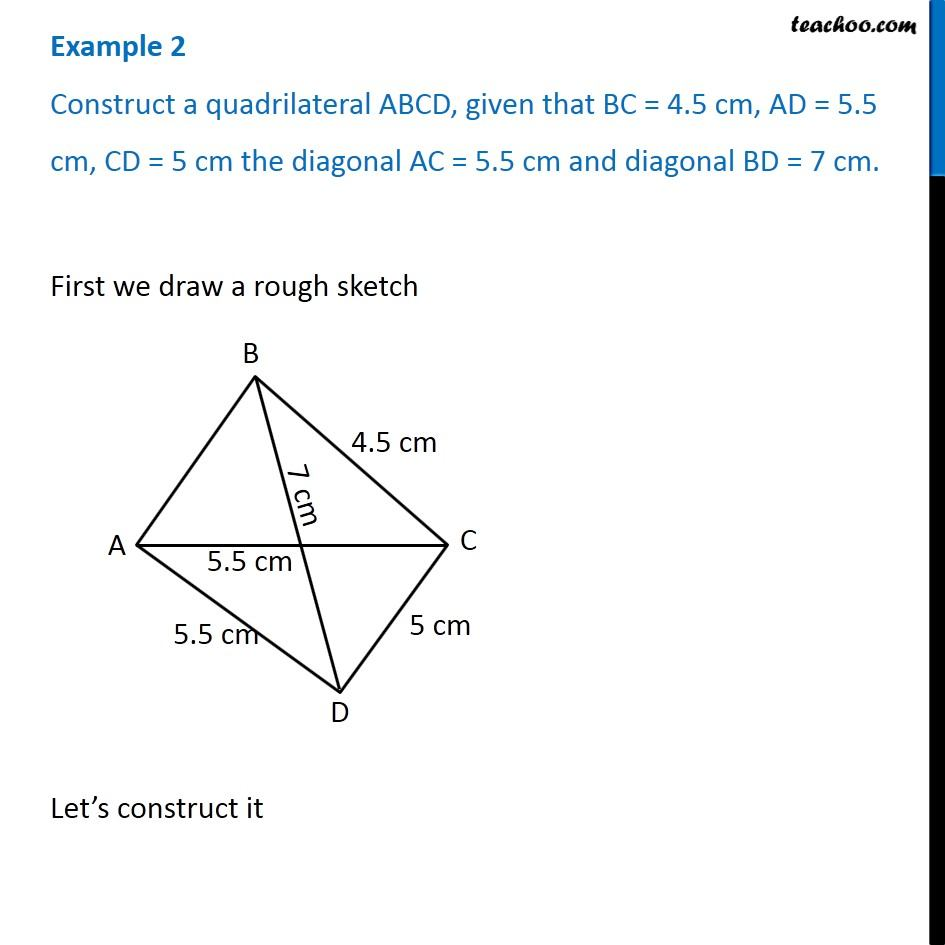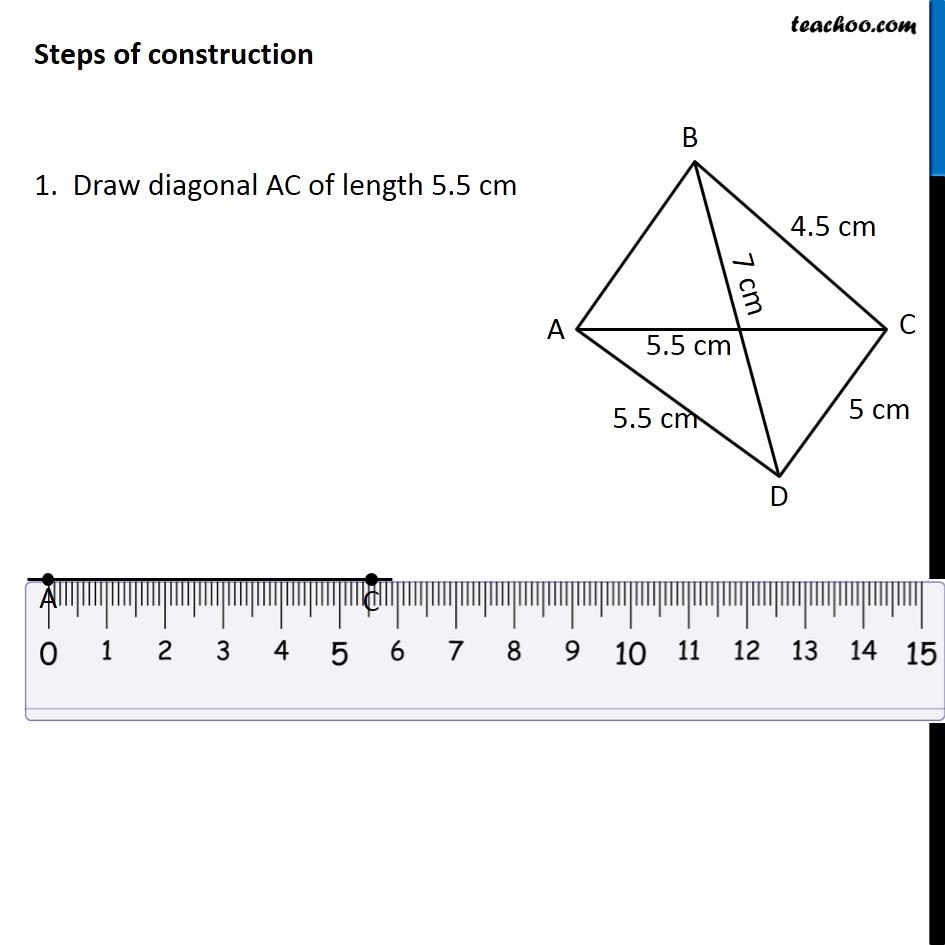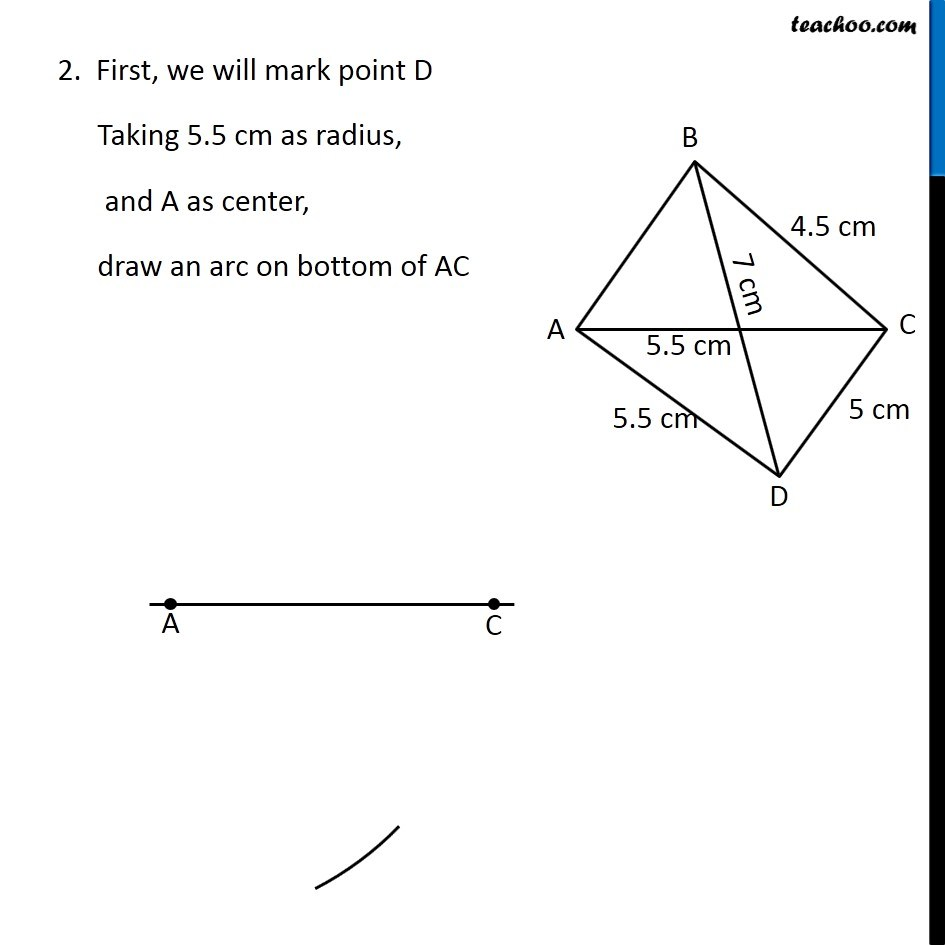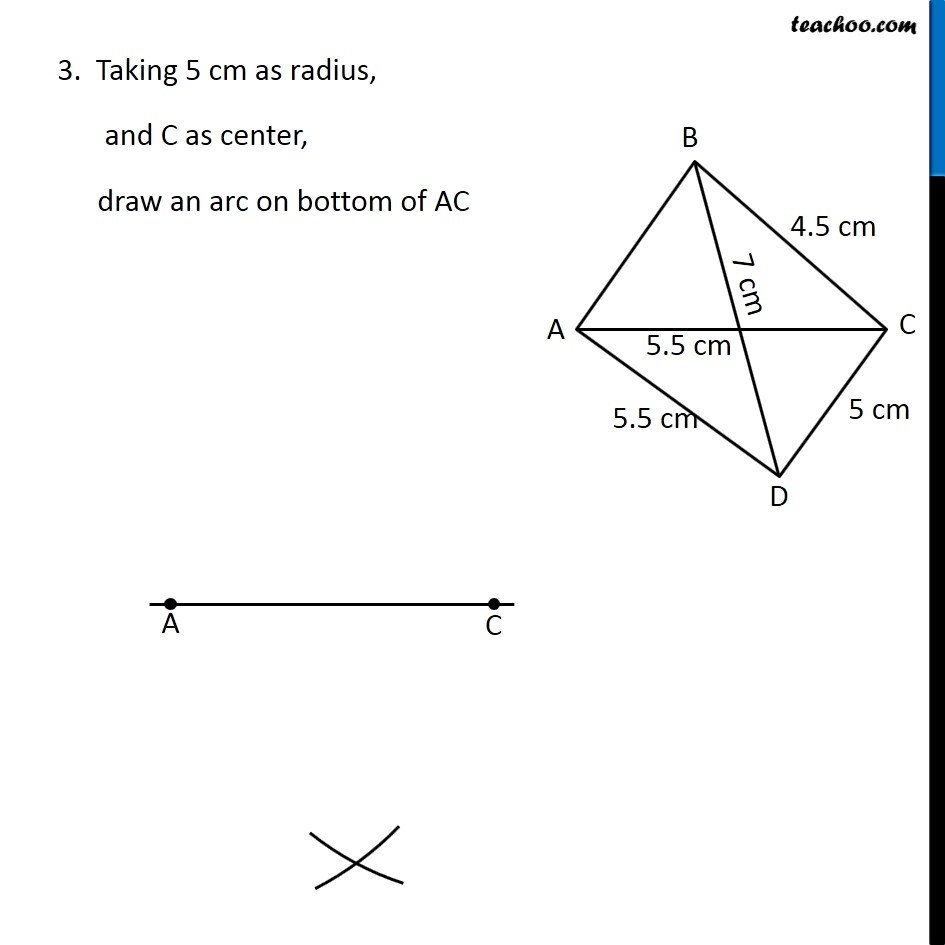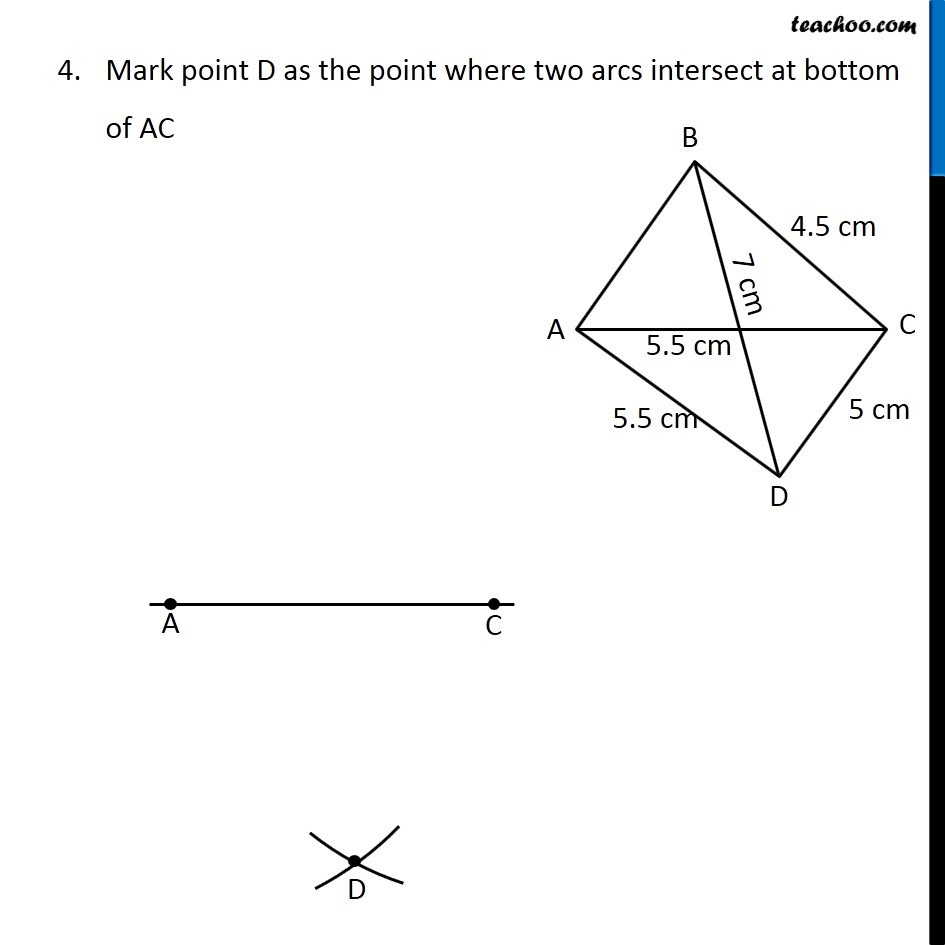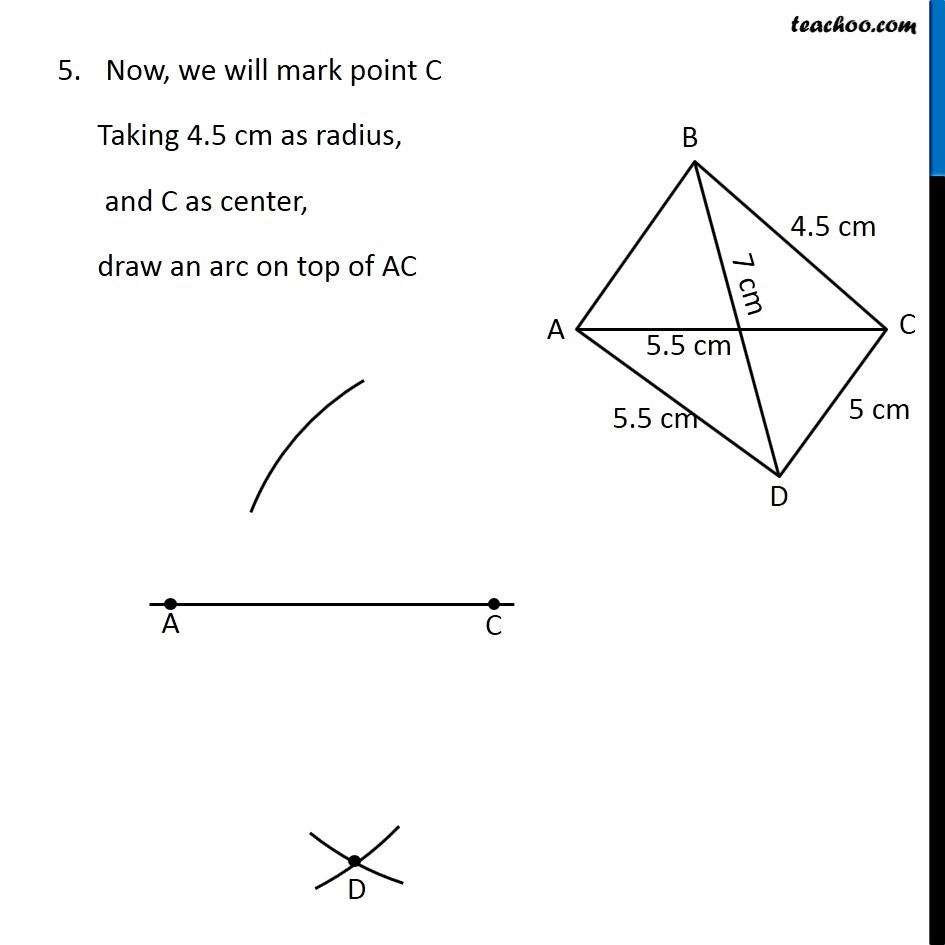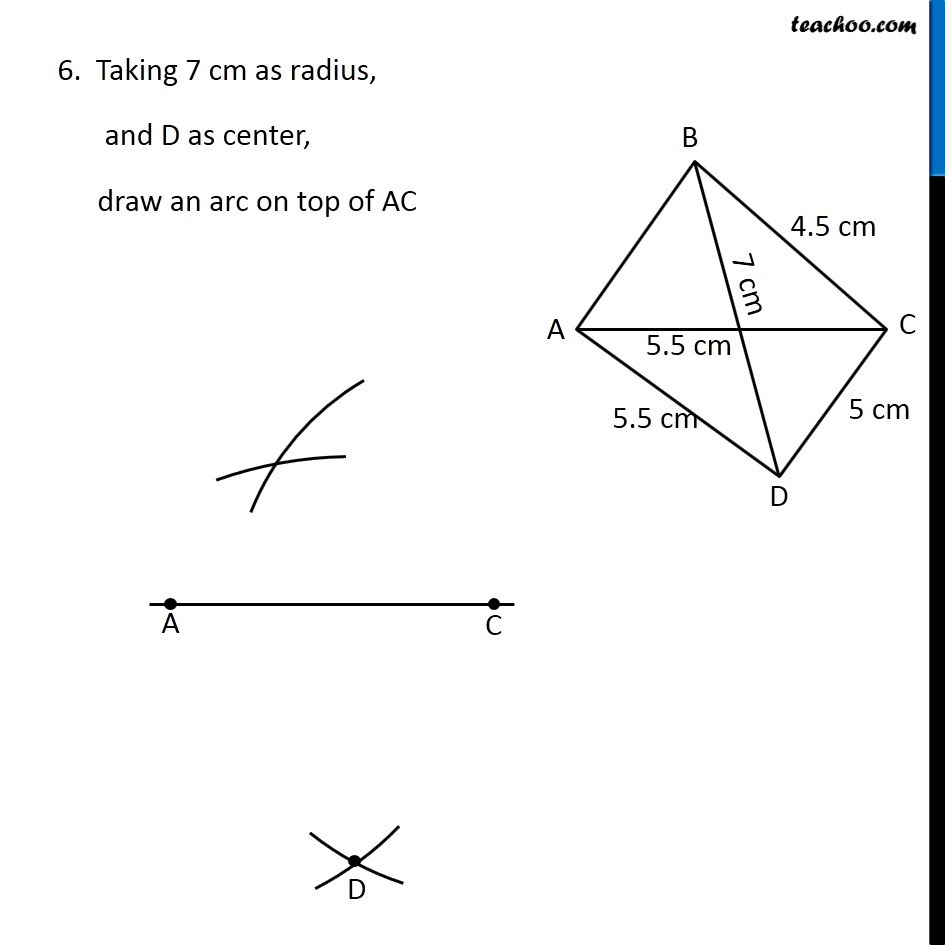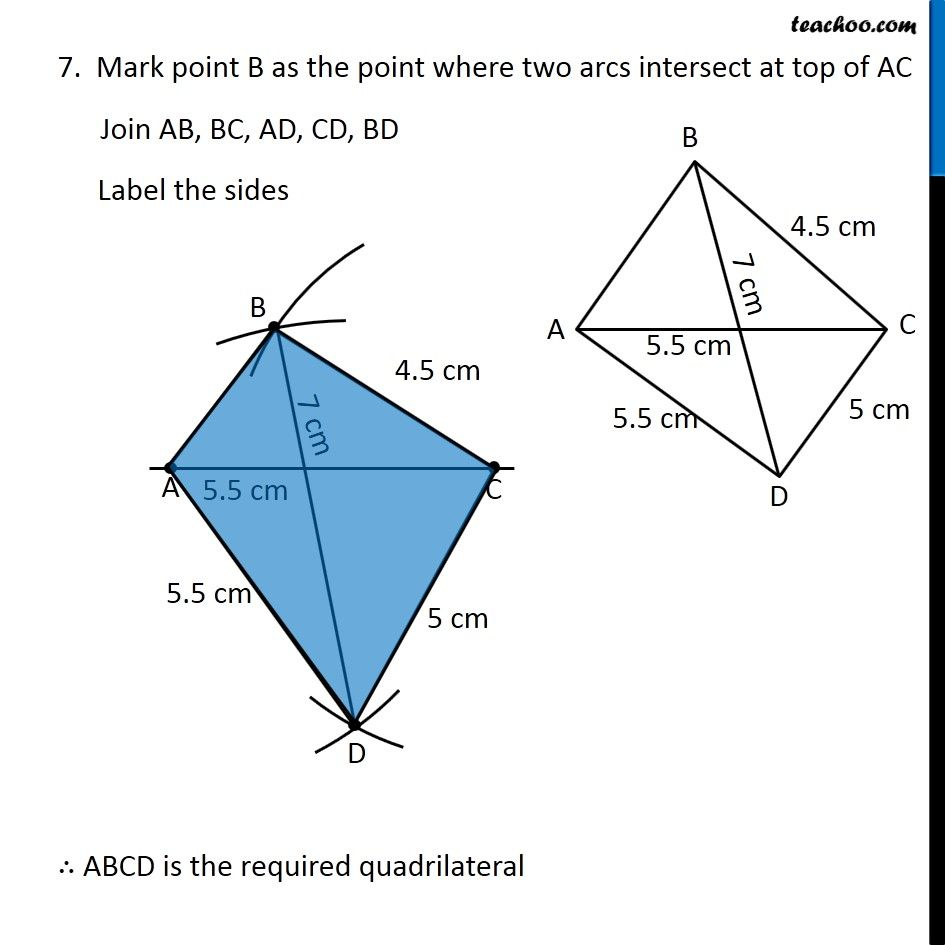Learn in your speed, with individual attention - Teachoo Maths 1-on-1 Class

### Transcript

Example 2 Construct a quadrilateral ABCD, given that BC = 4.5 cm, AD = 5.5 cm, CD = 5 cm the diagonal AC = 5.5 cm and diagonal BD = 7 cm. First we draw a rough sketch Let’s construct it Steps of construction 1. Draw diagonal AC of length 5.5 cm 2. First, we will mark point D Taking 5.5 cm as radius, and A as center, draw an arc on bottom of AC 3. Taking 5 cm as radius, and C as center, draw an arc on bottom of AC Mark point D as the point where two arcs intersect at bottom of AC Mark point D as the point where two arcs intersect at bottom of AC 6. Taking 7 cm as radius, and D as center, draw an arc on top of AC 7. Mark point B as the point where two arcs intersect at top of AC Join AB, BC, AD, CD, BD Label the sides